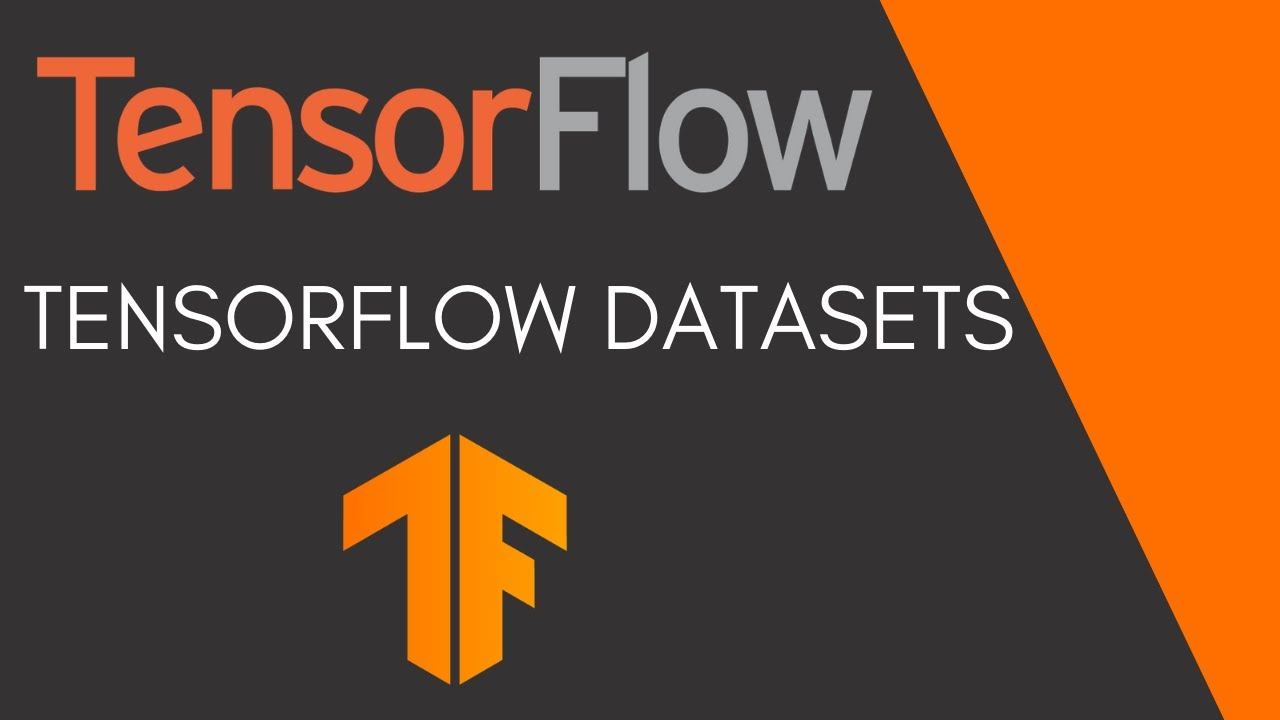# TensorFlow Tutorial 12 - TensorFlow DatasetsIn this video we look at the datasets that are available to us through TensorFlow Datasets (tfds) and how we load them and then doing preprocessing, shuffling, batching, prefetching etc.

In this video we look at the datasets that are available to us through TensorFlow Datasets (tfds) and how we load them and then doing preprocessing, shuffling, batching, prefetching etc. For the example we load an image dataset (mnist) and a text dataset (imdb) and create simple models to do image classification and sentiment analysis.

## What is TensorFlow? TensorFlow

An end-to-end open-source platform for Machine Learning. Before we start with TensorFlow, we will need to know what machine learning and deep learning technologies are.

## A TensorFlow Modeling Pipeline using TensorFlow Datasets and TensorBoard

This article investigates TensorFlow components for building a toolset to make modeling evaluation more efficient. Specifically, TensorFlow Datasets (TFDS) and TensorBoard (TB) can be quite helpful in this task.

## Keras vs. Tensorflow - Difference Between Tensorflow and Keras

Keras vs Tensorflow - Learn the differences between Keras and Tensorflow on basis of Ease to use, Fast development,Functionality,flexibility,Performance etc

## Deployment of a TensorFlow model to Production using TensorFlow Serving

Deploy a Deep Learning Model to Production using TensorFlow Serving.

## A TensorFlow Modeling using TensorFlow Datasets and TensorBoard

While completing a highly informative AICamp online class taught by Tyler Elliot Bettilyon (TEB) called Deep Learning for Developers, I …